# Knowledge Base

## Solution 34603: Finding the X-Intercept of a Function on the TI-83 Plus and TI-84 Plus Family of Graphing Calculators.

### How can I find the x-intercepts of a function on the TI-83 Plus and TI-84 Plus family of graphing calculators?

To find the x-intercepts of a function, the zero command must be used. Follow the example below for help with using the zero command.

Example: 2X - 7

1) Press [Y=] to access the Y= Editor.
2) Press [Clear] then  [X,T,θ,n] [-] 
3) Press [zoom] then  to graph the function and select standard viewing window.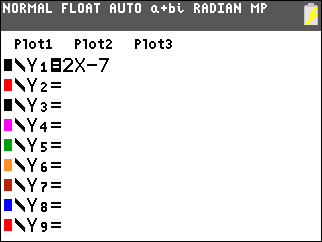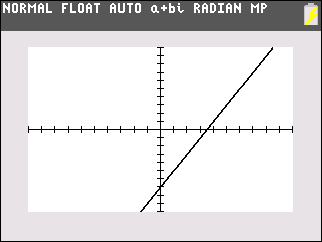4) Press [2nd] then [trace] to access the CALCULATE menu
5) Press  to select the zero command.
6) "Left Bound?" will be displayed, select a point on the graph that is to the left of the x-intercept by using the [←] or [→] keys.
7) Once selected press [enter] to mark this as the Left Bound.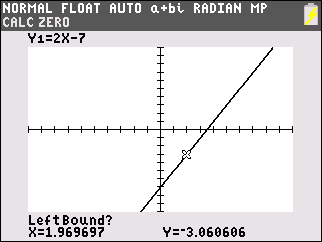8) "Right Bound?" will be displayed, select a point on the graph that is to the right of the x-intercept using the [←] or [→] keys.
9) Once selected press [enter] to mark this as the Right Bound.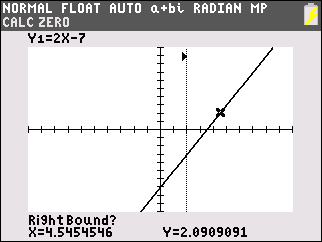10) "Guess?" will be displayed using the [←] or [→] keys, move the cursor until it is close to or directly on the x-intercept
11) Press [enter] to submit your Guess and display the exact point of the x-intercept which is (3.5,0)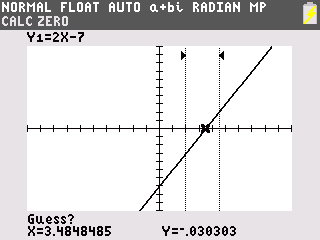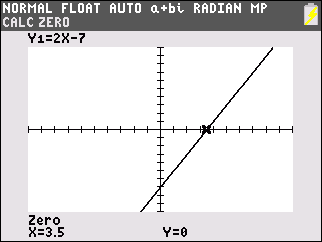Please see the TI-83 Plus and TI-84 Plus Family guidebooks for additional information.

Last updated: 7/24/2023